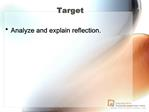Download PresentationTarget

# Target - PowerPoint PPT PresentationDownload Presentation## Target

- - - - - - - - - - - - - - - - - - - - - - - - - - - E N D - - - - - - - - - - - - - - - - - - - - - - - - - - -
##### Presentation Transcript

1. Target Analyze and explain reflection.

2. POD What is the Normal to a surface? It is a ray that is perpendicular to the surface.

3. Mirror Lab

4. Mirror Lab

6. Target Analyze and explain reflection.

7. POD

11. House

12. Target Analyze and explain reflection from curved mirrors.

13. Agenda Check HW 15.1 outline Measuring angles Quiz makeup Concave Mirror Worksheet

14. Measuring Angles Worksheet

15. Measuring Angles Worksheet

16. The light ray is approaching the first mirror at an angle of 45-degrees with the mirror surface. Trace the path of the light ray as it bounces off the mirror; continue tracing the ray until it finally exits from the mirror system. How many times will the ray reflect before it finally exits?

18. POD Determine where the image will be using rays.

19. POD Determine where the image will be using rays. Is the image real or virtual?

20. Reflection

21. Target Draw ray diagrams to show the path of light as it bounces off mirrors.

23. Reflection

25. Ray diagrams show the path that several light rays travel and can be used: For finding the focus To determine if image is magnified or reduced To see if the image is flipped over

34. POD Determine where the image will be using rays.

36. Targets Analyze and explain refraction How does refraction apply to lenses? Do different angles produce different effects? With respect to the normal, which way does light refract as it enters a lens? �as it leaves a lens?

37. Lens Lab�. Turn in corrections.